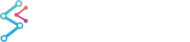﻿ The Digital (Step) Band Series Type | JavaScript Chart DocumentationSciChart.js JavaScript 2D Charts API > 2D Chart Types > The Digital (Step) Band Series Type
The Digital (Step) Band Series Type

A Digital Band Series, or High-Low Fill between two Digital or Step lines can be created using the FastBandRenderableSeries type.

The JavaScript Digital Band Chart Example can be found in the SciChart.Js Examples Suite on Github, or our live demo at demo.scichart.com

## Create a Digital Band Series

To create a Javascript Digital Band Chart with SciChart.js, use the following code:

JavaScript Line Chart
Copy Code
```import { XyyDataSeries } from "scichart/Charting/Model/XyyDataSeries";
import { NumericAxis } from "scichart/Charting/Visuals/Axis/NumericAxis";
import { FastBandRenderableSeries } from "scichart/Charting/Visuals/RenderableSeries/FastBandRenderableSeries";
import { SciChartSurface } from "scichart/Charting/Visuals/SciChartSurface";
import { NumberRange } from "scichart/Core/NumberRange";
import { EAxisAlignment } from "scichart/types/AxisAlignment";

// Create a SciChartSurface
const { wasmContext, sciChartSurface } = await SciChartSurface.create(divElementId);
sciChartSurface.xAxes.add(new NumericAxis(wasmContext, { axisAlignment: EAxisAlignment.Top }));
new NumericAxis(wasmContext, { axisAlignment: EAxisAlignment.Left, growBy: new NumberRange(0.4, 0.4) })
);
// The bandseries requires a special dataseries type called XyyDataSeries
// This stores X, Y1, Y2 point data for the two lines in the band
const dataSeries = new XyyDataSeries(wasmContext);
const POINTS = 1000;
const STEP = (3 * Math.PI) / POINTS;
for (let i = 0; i <= 1000; i++) {
const k = 1 - i / 2000;
dataSeries.append(i, Math.sin(i * STEP) * k * 0.7, Math.cos(i * STEP) * k);
}
// Create the band series and add to the chart
const rendSeries = new FastBandRenderableSeries(wasmContext, {
dataSeries,
strokeThickness: 2,
isDigitalLine: true.
});
rendSeries.fill = "#279B2733";
rendSeries.fillY1 = "#FF191933";
rendSeries.stroke = "#FF1919FF";
rendSeries.strokeY1 = "#279B27FF";
```

In the code above:

## Render a Gap in a Digital Band Series

It is possible to have null points or gaps in a Digital Band Series by passing a data point with a NaN value as the Y value. Please refer to the Common RenderableSeries Features article for more details.

Changing the above code as follows:

JavaScript Discontinuous Line Chart
Copy Code
```const dataSeries = new XyyDataSeries(wasmContext);
const POINTS = 1000;
const STEP = (3 * Math.PI) / POINTS;
for (let i = 0; i <= 1000; i++) {
const k = 1 - i / 2000;
dataSeries.append(i, i % 100 < 20 ? NaN : Math.sin(i * STEP) * k * 0.7, Math.cos(i * STEP) * k);
}
// Create the band series and add to the chart
const rendSeries = new FastBandRenderableSeries(wasmContext, { dataSeries, strokeThickness: 2, isDigitalLine: true });
```

Results in this: